# ML Wiki

## Decision Tree

This is a classification method used in Machine Learning and Data Mining that is based on Trees

### Rule-Based Classifiers

Suppose we have a set of rules

• if we group them by condition
• and then put redundant checks inside it
• we get a decision tree

Rules:

• IF pclass='1' AND sex='female' THEN survive=yes
• IF pclass='1' AND sex='male' AND age < 5 THEN survive=yes
• IF pclass='2' AND sex='female' THEN survive=yes
• IF pclass='2' AND sex='male' THEN survive=no
• IF pclass='3' AND sex='male' THEN survive=no
• IF pclass='3' AND sex='female' AND age < 4 THEN survive=yes
• IF pclass='3' AND sex='female' AND age >= 4 THEN survive=no

If we put if conditions inside

• IF pclass='1' THEN
• IF sex='female' THEN survive=yes
• IF sex='male' AND age < 5 THEN survive=yes
• IF pclass='2'
• IF sex='female' THEN survive=yes
• IF sex='male' THEN survive=no
• IF pclass='3'
• IF sex='male' THEN survive=no
• IF sex='female'
• IF age < 4 THEN survive=yes
• IF age >= 4 THEN survive=no

We have a decision tree:

•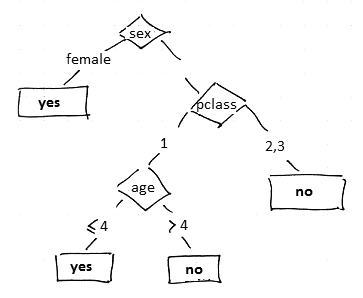### Decision Trees

Consider this dataset

Tid Refund Marital Status Income Cheat
1 Yes Single 125K No
2 No Married 100K No
3 No Single 70K No
4 Yes Married 120K No
5 No Divorced 95K Yes
6 No Married 60K No
7 Yes Divorced 220K No
8 No Single 85K Yes
9 No Married 75K No
10 No Single 90K Yes

There could be several decision trees for this dataset:

•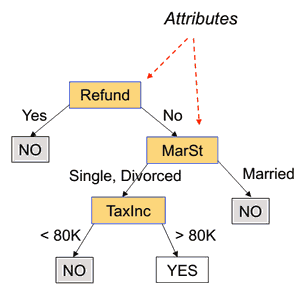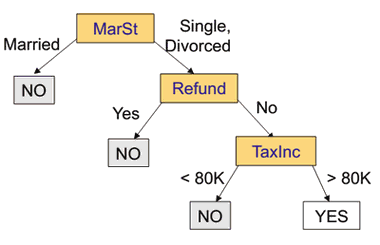Decision Tree:

• leaves are labels with the predicted class
• internal nodes are labeled with attributes used to decide which path to take
• edges are labeled with values for this attributes or with boolean tests

Classification:

• suppose we take a previously unseen records (11, no, married, 112k, ?)
• we need to predict a class for this label
• we put this instance on top of the tree and go the way to the leaf
• the leaf we end up is our class - cheat=no

### Error Measures

A tree is perfect if it makes no errors on the training set

•• this tree is perfect w.r.t. the training dataset
• learning error = 0%

Error measures:

• learning error: # of errors on the training dataset
• testing error: # of errors on the test set

## Learning Algorithms

There are several learning algorithms

• CART
• ID3, C4.5
• SLIQ, SPRINT, etc.

### Main Principles

(ID3 algo)

Given:

• training set $D$

The structure is recursive:

• let $D_t$ be a set of records that reach a node $t$
• at the beginning $t = \text{root}$ and $D_t \equiv D$
• if all $d \in D_t$ belong to the same class $y_t$
• then $t$ is labeled by $y_t$
• if $D_t \equiv \varnothing$
• then $t$ is labeled with the default class (e.g. the majority class)
• if $d \in D_t$ belong to different classes
• split $D_t$ into subsets $D_{t+1}$ and recursively apply the procedure to each

### Splitting

• there is huge # of ways to split a set
• how to determine the best split?

Splitting:

•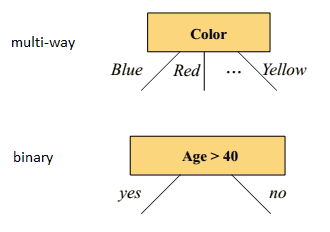• multiway: good for nominal, most of the time select all of the attributes
• binary: good for numeric, but need to find where to split

Splitting numerical attributes

• goal: want to find subsets that are more "pure" than the original one
• "pure" = degree of homogeneity
• $\fbox{$C_1$: 5,$C_2$: 5}$ - homogeneous, high degree of impurity
• $\fbox{$C_1$: 9,$C_2$: 1}$ - non-homogeneous, low degree of impurity
• the lower the better
• use Information Gain for that

### Stopping Conditions

Stop when

• all records in node $k$ belong to the same class
• the information gain is lower than some given threshold

## Information Gain

Given a set $S$ with $K$ classes $C_1, ..., C_K$

• let $p_k = \cfrac{| C_k |}{| C |}$ - probability of record belonging to class $k$
• $I(S)$ - is a function of impurity that we want to minimize

### Measures of Impurity

• Misclassification Error:
• $I(S) = 1 - \max_k p_k$
• Entropy and Information Gain
• $I(S) = - \sum_k p_k \cdot \log_2 p_k$
• Gini index:
• $I(S) = 1 - \sum_k p_k \cdot c_k$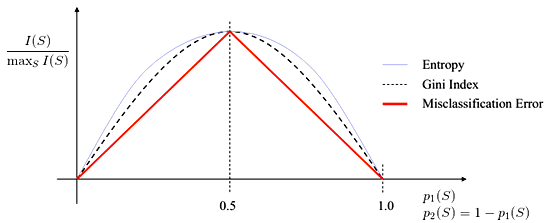### Information Gain

• how much information you get when partition the data?
• def: $\Delta I = I(S) - p_L \cdot I(S_L) - p_R \cdot I(S_R)$
• $p_L = \cfrac{| S_L |}{| S |}, p_R = \cfrac{| S_R |}{| S |}$
• $I$ - a measure of impurity

It's easy to generalize IG to Multi-way

• split $S$ to $K$ subsets $S_1, ..., S_K$
• $\Delta I = I(S) - \sum_k p_k \cdot I(S_k)$
• $\sum_k p_k \cdot I(S_k)$ is the Expected Value of Entropy in the partitioned dataset
• $E \big[ I \big( \{ S_1, ..., S_K \} \big) \big] = \sum_k p_k I(S_k) = - \sum_k p_k \log_2 p_k$
• with $p_k = \cfrac{| S_k |}{| S |}$
• but if we minimize this $\Delta I$, we end up with $K = N$
• i.e. we obtain as many subsets as there are records - and a set with just one element is 100% pure
• use Gain Ratio impurity
• $- \Delta I_K = \cfrac{\Delta I}{- \sum_k p_k \cdot \log_2 p_k}$

#### Example

Suppose we have a node with $S \equiv \fbox{$C_1$: 20,$C_2$: 30}$

• $S_L \equiv \fbox{$C_1$: 15,$C_2$: 5}$ and $S_L \equiv \fbox{$C_1$: 5,$C_2$: 25}$
• let $I$ be the Entropy function
• $I(S) = - \cfrac{20}{50} \log_2 \cfrac{20}{50} - \cfrac{30}{50} \log_2 \cfrac{30}{50} \approx 0.971$
• $I(S_L) = - \cfrac{15}{20} \log_2 \cfrac{15}{20} - \cfrac{5}{20} \log_2 \cfrac{5}{20} \approx 0.811$
• $I(S_R) = - \cfrac{5}{30} \log_2 \cfrac{5}{30} - \cfrac{5}{30} \log_2 \cfrac{5}{30} \approx 0.65$
• $\Delta I = I(S) - p_L \cdot I(S_L) - p_R \cdot I(S_R) = 0.971 - 0.4 \cdot 0.811 - 0.6 \cdot 0.65 = 0.26$

Another example with $S \equiv \fbox{$C_1$: 20,$C_2$: 30}$

• suppose that now we want to split to $S_L \equiv \fbox{$C_1$: 10,$C_2$: 15}$ and $S_L \equiv \fbox{$C_1$: 10,$C_2$: 15}$
• in this case it's clear that we don't have any IG
• so min value is 0, and there's no max boundary

### Splitting Algorithm

for each attribute $A_i$

• find the best partitioning $P^*_{A_i} = \{S_1, ..., S_K\}$ of $S$
• $P^*_{A_i}$ maximizes the information gain
• among all $\{ P^*_{A_i} \}$ select the maximal one
• split by it

Best to split in halves: $K = 2$

• how to choose $\alpha$ that splits $S$ into $S_L$ and $S_R$?
• try several, pick up the best
• note that $\Delta I$ is not monotonic - have to try all of them

## Overfitting

Perfect decision trees perform 100% accurate on the training set

• but perform very poorly on a test set
• this is called Overfitting
• when performing Machine Learning Diagnosis we see that the more nodes a decision tree has, the poorer it performs
•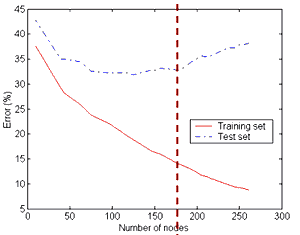How to get the optimal complexity of a tree?

• Pre-pruning
•  Stop before a tree becomes perfect (fully-grown)
•  Post-pruning
• Grow a perfect tree
•  Prune the nodes bottom-up

### Pre-Pruning

It's about finding a good stopping criterion

Simple ones:

• when # of instances in a node goes below some threshold
• when the misclassification error is lower than some threshold $\beta$
• $1 - \max_k p_k < \beta$
• when expanding current node doesn't give a significant information gain $\Delta I$
• $\Delta I < \beta$

More complex:

### Post-Pruning

• by Breiman, Olshen, Stone (1984)

## Practical Issues

### Decision Boundaries

the decision boundaries are rectilinear

•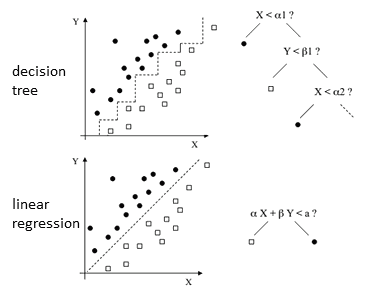• for some problems not always good
• use another models: Linear Regression, Neural Networks etc

### Handling Missing Values

Two options

• modify the algorithm

#### Algorithm Modification

Modification:

• suppose that we have some splitting test criterion $T$ and dataset $S$
• information gain for splitting $S$ using $T$ is
• $\Delta I (S, T) = I(S) - \sum_k \alpha_{T, k} \cdot I(S_k)$
• let $S_0 \subseteq S$ for which we can't evaluate $T$ (i.e. because needed values are missing)
• if $S_0 \not \equiv \varnothing$
• calculate the information gain as
• $\cfrac{|S - S_0|}{| S |} \Delta I (S - S_0, T)$
• suppose such $T$ is chosen, what to do with values from $S_0$?
• add them to all the subsets with weight proportional to the size of these subsets
• $w_k = \cfrac{| S |}{|S - S_0|}$
• and information gain is computed using sums of weights instead of counts

#### Example

X Y Z Class
a 70 Yes +
a 90 Yes -
a 85 No -
a 95 No -
a 70 No +
? 90 Yes +
b 78 No +
b 65 Yes +
b 75 No +
c 80 Yes -
c 70 Yes -
c 80 No +
c 80 No +
c 96 No +
• There is one missing value for $X$: $(?, 90, \text{Yes}, +)$
• let $I$ be the misclassification error
• $I(S - S_0) = 5/13$ (5 in "-", 8 in "+")
• $I(S - S_0 | X = a) = 2/5$
• $I(S - S_0 | X = b) = 0$
• $I(S - S_0 | X = c) = 2/5$
• calculate IG $\cfrac{|S - S_0|}{| S |} \Delta I (S - S_0, T)$
• $\Delta I = \cfrac{13}{14} \cdot (\cfrac{5}{13} - \cfrac{5}{13} \cdot \cfrac{2}{5} - \cfrac{3}{13} \cdot 0 - \cfrac{5}{13} \cdot \cfrac{2}{5}) = \cfrac{1}{14}$

Distribute the values to subsets

• according to the value of $X$
• but the rows with missing values are put to all the subsets
• and the weight is proportional to the size of this subset prior to adding these rows
 $Y$ $Z$ Class $w$ 70 Yes + 1 90 Yes - 1 85 No - 1 95 No - 1 70 No + 1 90 Yes + 5/13
 $Y$ $Z$ Class $w$ 78 No + 1 65 Yes + 1 75 No + 1 90 Yes + 3/13
 $Y$ $Z$ Class $w$ 80 Yes - 1 70 Yes - 1 80 No + 1 80 No + 1 96 No + 1 90 Yes + 5/13

#### Classification with Modification

Classification:

• let $P(C | E,T)$ be the probability of classifying case $E$ to class $C$ using tree $T$
• define it recursively:
• if $t = \text{root}(T)$ is a leaf (i.e. it's a singleton tree)
• then P(C | E,T) is the relative frequency of training cases in class $C$ that reach $T$
• if $t = \text{root}(T)$ is not a leaf and $t$ is partitioned using attribute $X$
• if $E.X = x_k$
• then $P(C | E,T) = P(C | E,T_k)$ where $T_k$ is a subtree of $T$ where $X = x_k$
• if $E.X$ is unknown,
• then $P(C|E,T) = \sum_{k=1}^{K} \cfrac{|S_k|}{|S-S_0|} \cdot P(C | E,T)$
• so we sum up probabilities of belonging to class $C$ from each child of $t$
• predict that a record belongs to class $C$ by selecting the highest probability $P(C|E,T)$

#### Example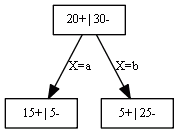• assume that $X$ is unknown - how to classify the case?
• $P(+ |E,T) = \sum_{k=1}^{K} P(+ | E,T_k) = \cfrac{20}{50} \cdot \cfrac{15}{20} + \cfrac{30}{50} \cdot \cfrac{5}{30} = \cfrac{20}{50}$
• $P(- |E,T) = \sum_{k=1}^{K} P(- | E,T_k) = \cfrac{20}{50} \cdot \cfrac{5}{20} + \cfrac{30}{50} \cdot \cfrac{25}{30} = \cfrac{30}{50}$
• $P(- |E,T) > P(+ |E,T) \Rightarrow$ predict "$-$"

## Decision Tree Learning Examples

### Example 1

Suppose we have the following data:

•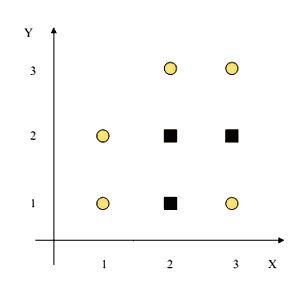• 2 classes: $\square$ (S) and $\bigcirc$ (C)
• there are 5S and 3C, total 8:
• $I(S) = - \frac{3}{8} \log_2 \frac{3}{8} - \frac{5}{8} \log_2 \frac{5}{8} = 0.954$
• there are 2 attributes: $X$ and $Y$
• and 2 ways to split each: $X < 1.5, X < 2.5, Y < 1.5, Y < 2.5$
$S_L$ $I(S_L)$ $S_R$ $I(S_R)$ $E[I(\{S_L, S_R\})]$ $\Delta I$
$X < 1.5$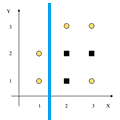$2C$ 0 $3C, 3S$ 1 $\frac{2}{8} \cdot 0 + \frac{5}{8} \cdot 1 = 0.75$ $0.954 - 0.75 = \fbox{0.204}$
$X < 2.5$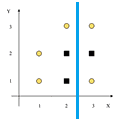$3C, 2S$ 0.971 $2C, 1S$ 0.918 $\frac{5}{8} \cdot 0.971 + \frac{3}{8} \cdot 0.918 = 0.951$ 0.003
$Y < 1.5$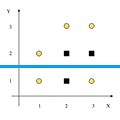$2C, 1S$ 0.918 $3C, 2S$ 0.971 0.951 0.003
$Y < 2.5$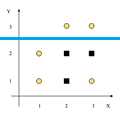$2C$ 1 $3C, 3S$ 0 0.75 0.204

We decide to use $X < 1.5$ to split the data

•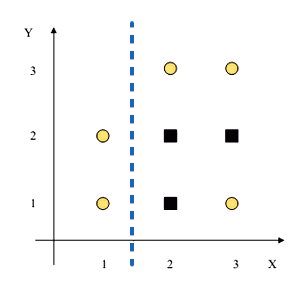• left part is pure - no need to split it
• right part: $3C, 3S$, it's not pure: $I(S) = 1$

$I(S_L)$ $I(S_R)$ $\Delta I$
$X < 2.5$ 0.918 0.918 0.082
$Y < 1.5$ 1 1 0
$Y < 2.5$ 0.811 0 $\fbox{0.459}$

• $Y < 2.5$ - next best splitting criterion
•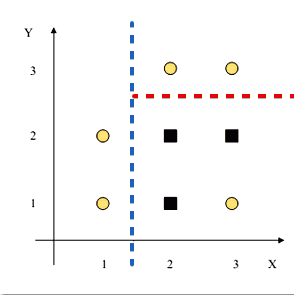• if we stop here, we obtain the following tree:
•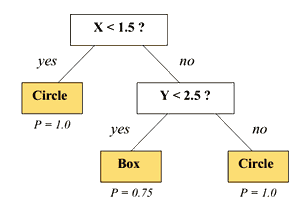• this tree is not a perfect tree - we can continue and at the end obtain a perfect one

## Pros and Cons

• fast
• handles both symbolic and numerical attributes
• works well with redundant attributes
• invariant to any monotonic transformation of the dataset
• robust to Outliers
• e.g. a tree with $\text{income}$ will behave the same way as a tree with $\sqrt{\text{income}}$
• easy to interpret and explain to non-specialists
• help to understand what are most important attributes